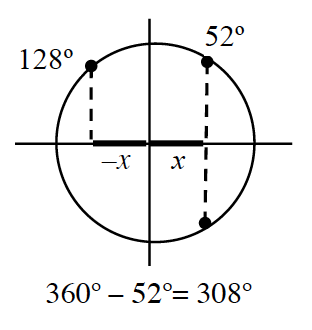### Home > INT3 > Chapter 12 > Lesson 12.1.2 > Problem12-26

12-26.

Salina, Tamara, and Uma are working on their homework together. Salina tells her friends that she has $θ = 52^\circ$ for the inverse cosine problem she is working on and asks if they have the same answer. Tamara says she has $θ = 128^\circ$, and Uma volunteers her answer of $θ = 308^\circ$. Is it possible that these are all solutions to the same problem? Justify your answer.

Picture a unit circle. Is it possible for the cosine of three different angles in three different quadrants to be the same?

Use the unit circle below to help you. Is cosine the same for all of these angles?